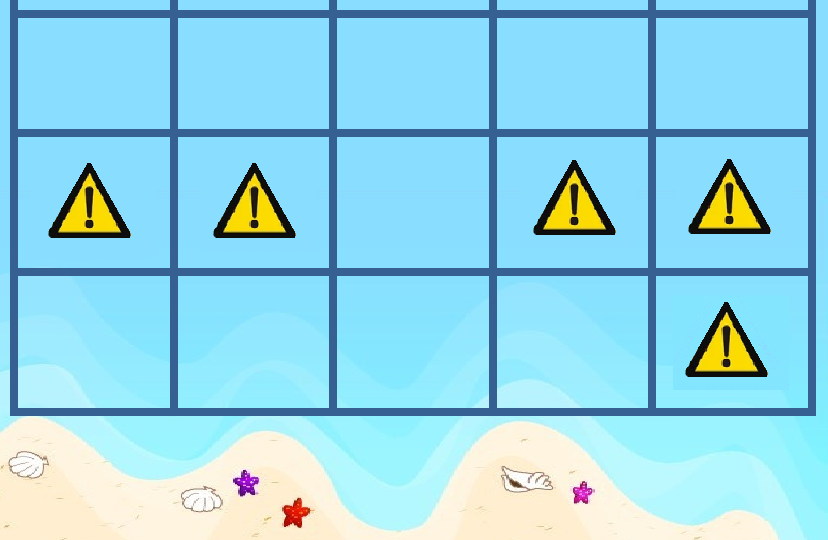## 【NOI2017】泳池

### 题目描述

• 必须保证安全性。即游泳场中的每一个单位海域都是安全的。

• 必须是矩形。即游泳场必须是整个网格中的一个 $a \times b$ 的子网格。

• 必须和海滩相邻。即游泳场的下边界必须紧贴网格的下边界。### 提示

$x^{p-1} \equiv 1 \mod p$，其中 $p$ 为质数，$x \in [1,p)$。

### 数据范围

3$\leq 10$$\leq 8 4\leq 10$$\leq 9$
5$\leq 10$$\leq 10 6\leq 1000$$\leq 7$
7$\leq 1000$$\leq 8 8\leq 1000$$\leq 9$
9,10,11$\leq 1000$$\leq 100 12,13,14\leq 1000$$\leq 1000$
15,16$\leq 10^9$$\leq 10 17,18\leq 10^9$$\leq 100$
19,20$\leq 10^9$$\leq 1000$

### 题目来源

NOI 2017 Day 1

#### 关于提交的说明

Judge Duck Online | 评测鸭在线
Server Time: 2022-06-26 02:02:43 | Loaded in 0 ms | Server Status